# Wang-Landau method

The Wang-Landau method was proposed by F. Wang and D. P. Landau   to compute the density of states,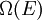$\Omega (E)$, of Potts models; where$\Omega(E)$ is the number of microstates of the system having energy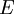$E$.

## Outline of the method

The Wang-Landau method, in its original version, is a simulation technique designed to achieve a uniform sampling of the energies of the system in a given range. In a standard Metropolis Monte Carlo in the canonical ensemble the probability of a given microstate,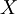$X$, is given by: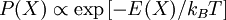$P(X) \propto \exp \left[ - E(X)/k_B T \right]$;

whereas for the Wang-Landau procedure one can write: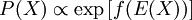$P(X) \propto \exp \left[ f(E(X)) \right]$ ;

where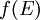$f(E)$ is a function of the energy.$f(E)$ changes during the simulation in order produce a predefined distribution of energies (usually a uniform distribution); this is done by modifying the values of$f(E)$ to reduce the probability of the energies that have been already visited, i.e. If the current configuration has energy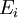$E_i$,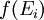$f(E_i)$ is updated as: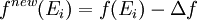$f^{new}(E_i) = f(E_i) - \Delta f$ ;

where it has been considered that the system has discrete values of the energy (as happens in Potts Models), and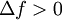$\Delta f > 0$.

Such a simple scheme is continued until the shape of the energy distribution approaches the one predefined. Notice that this simulation scheme does not produce an equilibrium procedure, since it does not fulfill detailed balance. To overcome this problem, the Wang-Landau procedure consists in the repetition of the scheme sketched above along several stages. In each subsequent stage the perturbation parameter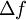$\Delta f$ is reduced. So, for the last stages the function$f(E)$ hardly changes and the simulation results of these last stages can be considered as a good description of the actual equilibrium system, therefore: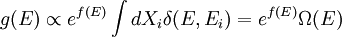$g(E) \propto e^{f(E)} \int d X_i \delta( E, E_i ) = e^{f(E)} \Omega(E)$;

where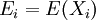$E_i = E(X_i)$,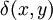$\delta(x,y)$ is the Kronecker Delta, and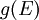$g(E)$ is the fraction of microstates with energy$E$ obtained in the sampling.

If the probability distribution of energies,$g(E)$, is nearly flat (if a uniform distribution of energies is the target), i.e.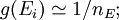$g(E_i) \simeq 1/n_{E} ;$; for each value$E_i$ in the selected range,

with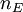$n_{E}$ being the total number of discrete values of the energy in the range, then the density of states will be given by: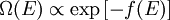$\Omega(E) \propto \exp \left[ - f(E) \right]$

### Microcanonical thermodynamics

Once one knows$\Omega(E)$ with accuracy, one can derive the thermodynamics of the system, since the entropy in the microcanonical ensemble is given by: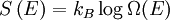$S \left( E \right) = k_{B} \log \Omega(E)$

where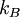$k_{B}$ is the Boltzmann constant.

## Extensions

The Wang-Landau method has inspired a number of simulation algorithms that use the same strategy in different contexts. For example:

### Phase equilibria

In the original version one computes the entropy of the system as a function of the internal energy,$E$, for fixed conditions of volume, and number of particles. In Refs.  it was shown how the procedure can be applied to compute other thermodynamic potentials that can be subsequently used to locate phase transitions. For instance, one can compute the Helmholtz energy function ,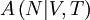$A \left( N | V, T \right)$ as a function of the number of particle$N$ for fixed conditions of volume,$V$, and temperature,$T$.

### Refinement of the results

It can be convenient to supplement the Wang-Landau algorithm, which does not fulfil detailed balance, with an equilibrium simulation . In this equilibrium simulation one can use the final result for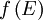$f\left( E \right)$ (or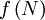$f\left( N \right)$) extracted from the Wang-Landau technique as a fixed function to weight the probability of the different configurations. Such a strategy simplifies the estimation of error bars, provides a good test of the results consistency, and can be used to refine the numerical results.

## Applications

The Wang-Landau algorithm has been applied successfully to several problems in physics[?] , biology[?] , and chemistry[?] .